# Nominal Interest Rate### Written byTrue Tamplin, BSc, CEPF® | Reviewed by Editorial Team

Updated on December 29, 2022

A nominal interest rate is the theoretical or stated interest rate on an investment, usually expressed as a percentage of the principal amount.

The nominal interest rate doesn't take into account inflation and other factors that will erode the purchasing power of the investment over time.

## Why Does the Nominal Interest Rate Matter?

The nominal interest rate is the starting point for most investment decisions and analyses.

Investors look at the possible gains against their risk profile, calculate a required return based on that information, and then compare it to available investments.

## How to Calculate the Nominal Interest Rate

There isn't one single formula used for determining what an individual's or company's Nominal Interest Rate is. One can calculate a nominal interest rate by using this equation: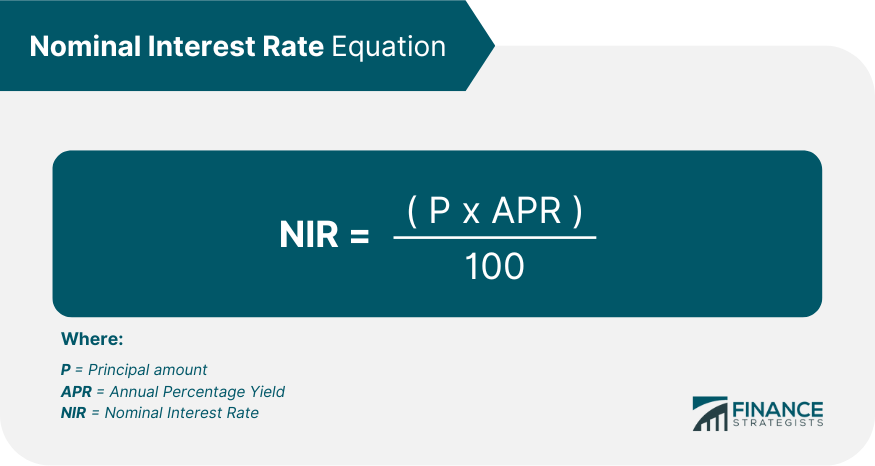## Example on How to Use a Nominal Interest Rate in an Investment Analysis

For example, if someone is looking to invest \$100 with an annual interest rate of 12%, they would calculate the nominal interest rate as follows: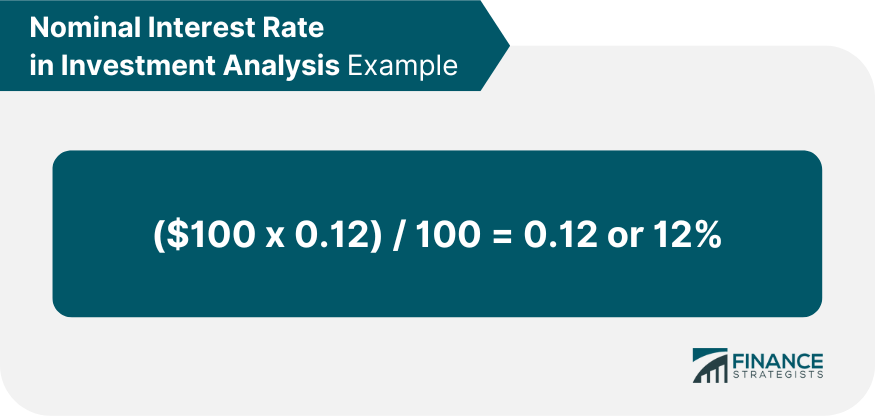## Difference Between Nominal Interest Rate and Real Interest Rate

The real interest rate takes into account inflation or deflation of purchasing power over time (the price level) whereas the Nominal Interest Rate does not factor this in.

## The Fisher Effect

Well-known economist Irving Fisher coined the Fisher Effect which is a theory that describes the relationship between nominal and real interest rates.

It states that the real interest rate of a savings account is actually the difference between the nominal interest rate and the expected inflation rate.

In an equation, it can be illustrated as: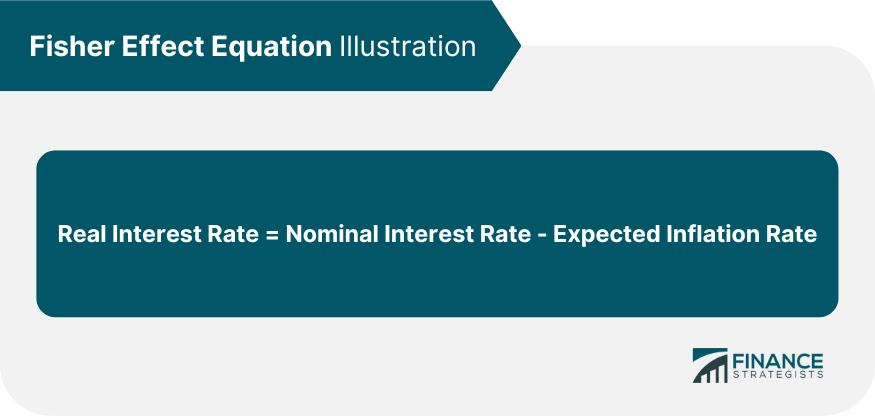Furthermore, the nominal interest rate can also be calculated through this formula: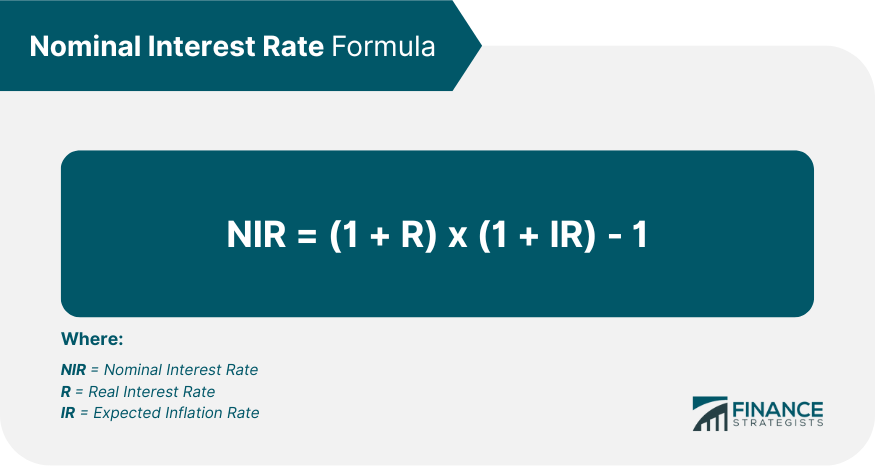### Fisher Effect Sample Illustration

For example, a certain savings account carries a real interest rate of 5% at an expected inflation rate of 3%.

To use the first equation under the Fisher Effect, we find that: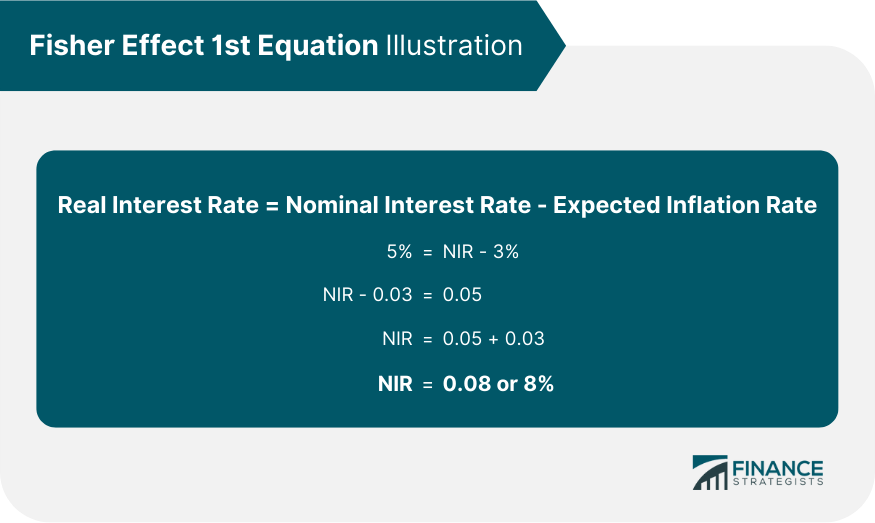Using the second equation under the Fisher Effect: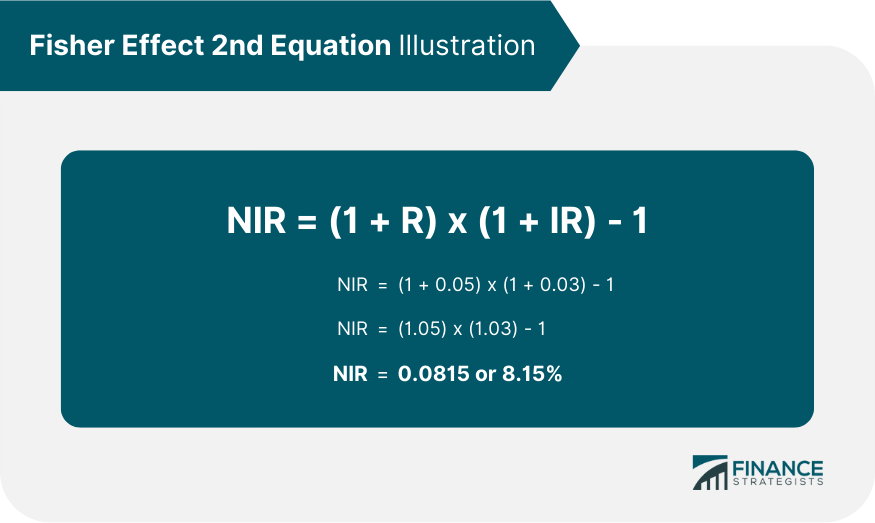Both equations produce an approximately similar result with the nominal interest rate at 8-8.15%

## Implication on the Use of Nominal Interest Rate

The nominal interest rate is just one component of any investment decision and should never be used in isolation to make decisions about an investment, particularly given that inflation exists over time.

The Fisher Effect helps provide clarity into understanding how real rates are determined based on expected future inflation levels.

## Nominal Interest Rate FAQs

### What is nominal interest rate?

A nominal interest rate is the theoretical or stated interest rate on an investment, usually expressed as a percentage of the principal amount. The nominal interest rate doesn't take into account inflation and other factors that will erode the purchasing power of the investment over time.

### How does nominal interest rate differ from real interest rates?

A nominal interest rate is the starting point for most investment decisions and analysis. Investors look at the possible gains against their risk profile, calculate a required return based on that information and then compare it to available investments. The real (or inflation-adjusted) interest rate takes into account inflation or deflation of purchasing power over time (the price level) whereas nominal interest rate does not factor this in.

### How is nominal interest rate calculated?

The nominal interest rate can be found by using the following formula: (P * APR) / 100 = NIR where P = Principal amount, APR = Annual Percentage Yield and NIR = Nominal Interest Rate. If you are looking to invest \$100 with an annual interest rate of 12%, you would calculate the nominal interest rate as follows: (\$100 * .12) / 100 = .12 or 12%.

### Why do we need to know nominal interest rates and real interest rates?

In financial analysis, nominal returns are typically used as a starting point for comparison across different investments. Inflation is often not taken into account when considering nominal returns since the intention of most investors is capital appreciation rather than preserving purchasing power or inflation-adjusted income. For this reason, nominal interest rates and real interest rate calculations can be used to analyze nominal returns and determine if the investment is appropriate for an investor's risk profile.

### What is the Fisher Effect?

The Fisher Effect is a useful economic concept that helps to illustrate nominal interest rates. It provides insight into how real interest rate changes are affected by nominal interest rates and expected inflation levels.